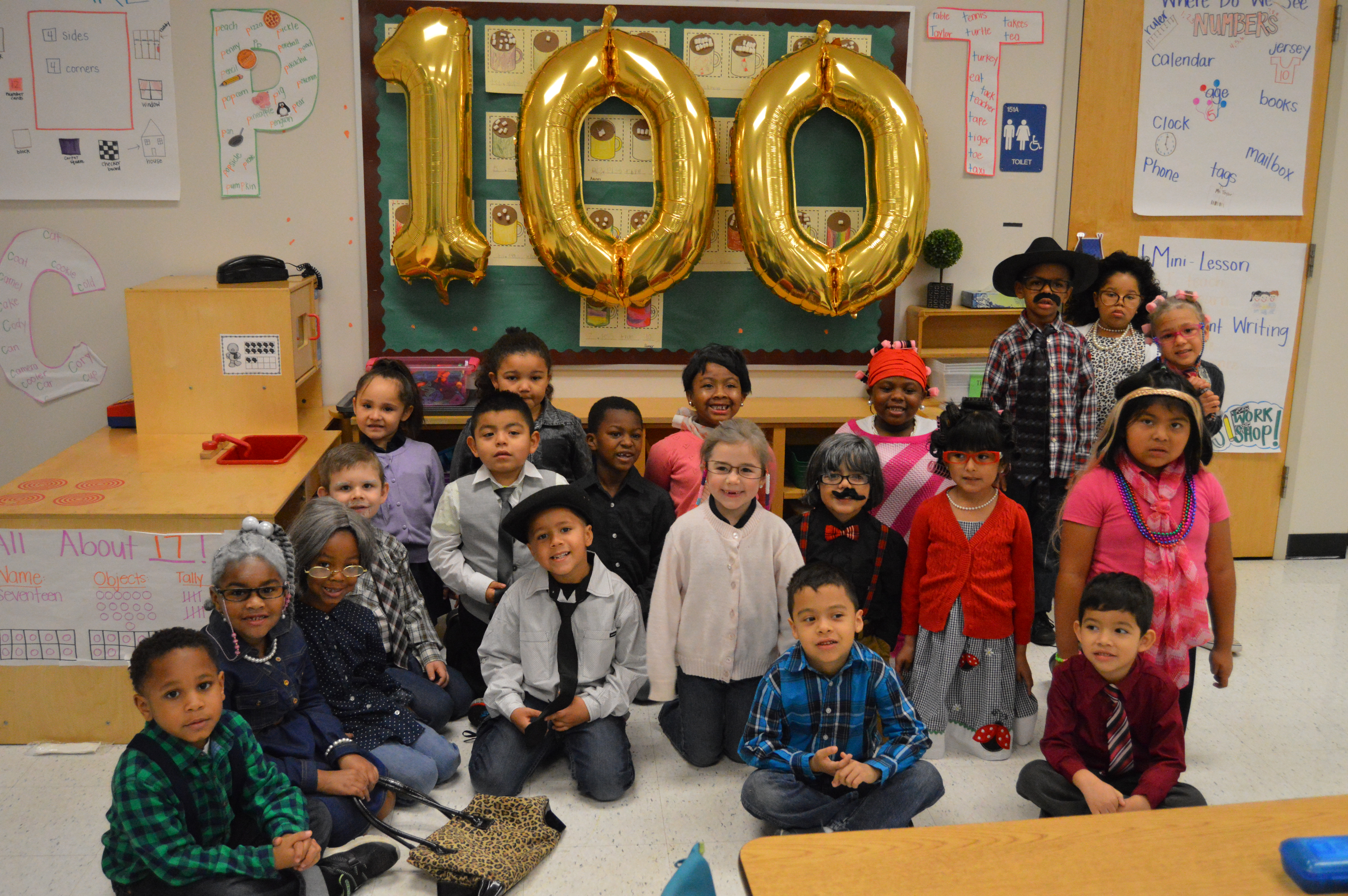# Test PageHere is some text. Here is some text. Here is some text. Here is some text. Here is some text. Here is some text. Here is some text. Here is some text. Here is some text. Here is some text. Here is some text. Here is some text. Here is some text. Here is some text. Here is some text. Here is some text. Here is some text. Here is some text. Here is some text. Here is some text. Here is some text. Here is some text. Here is some text. Here is some text. Here is some text. Here is some text. Here is some text. Here is some text. Here is some text. Here is some text. Here is some text. Here is some text. Here is some text. Here is some text. Here is some text. Here is some text. Here is some text. Here is some text. Here is some text.

Here is some text. Here is some text. Here is some text. Here is some text. Here is some text. Here is some text. Here is some text. Here is some text. Here is some text. Here is some text. Here is some text. Here is some text. Here is some text. Here is some text. Here is some text. Here is some text. Here is some text. Here is some text. Here is some text. Here is some text. Here is some text. Here is some text. Here is some text. Here is some text. Here is some text. Here is some text.

It is very easy to make buttons.

# Test PageHere is some text. Here is some text. Here is some text. Here is some text. Here is some text. Here is some text. Here is some text. Here is some text. Here is some text. Here is some text. Here is some text. Here is some text. Here is some text. Here is some text. Here is some text. Here is some text. Here is some text. Here is some text. Here is some text. Here is some text. Here is some text. Here is some text. Here is some text. Here is some text. Here is some text. Here is some text. Here is some text. Here is some text. Here is some text. Here is some text. Here is some text. Here is some text. Here is some text. Here is some text. Here is some text. Here is some text. Here is some text. Here is some text. Here is some text.

Here is some text. Here is some text. Here is some text. Here is some text. Here is some text. Here is some text. Here is some text. Here is some text. Here is some text. Here is some text. Here is some text. Here is some text. Here is some text. Here is some text. Here is some text. Here is some text. Here is some text. Here is some text. Here is some text. Here is some text. Here is some text. Here is some text. Here is some text. Here is some text. Here is some text. Here is some text.

It is very easy to make buttons.

# Test PageHere is some text. Here is some text. Here is some text. Here is some text. Here is some text. Here is some text. Here is some text. Here is some text. Here is some text. Here is some text. Here is some text. Here is some text. Here is some text. Here is some text. Here is some text. Here is some text. Here is some text. Here is some text. Here is some text. Here is some text. Here is some text. Here is some text. Here is some text. Here is some text. Here is some text. Here is some text. Here is some text. Here is some text. Here is some text. Here is some text. Here is some text. Here is some text. Here is some text. Here is some text. Here is some text. Here is some text. Here is some text. Here is some text. Here is some text.

Here is some text. Here is some text. Here is some text. Here is some text. Here is some text. Here is some text. Here is some text. Here is some text. Here is some text. Here is some text. Here is some text. Here is some text. Here is some text. Here is some text. Here is some text. Here is some text. Here is some text. Here is some text. Here is some text. Here is some text. Here is some text. Here is some text. Here is some text. Here is some text. Here is some text. Here is some text.

It is very easy to make buttons.